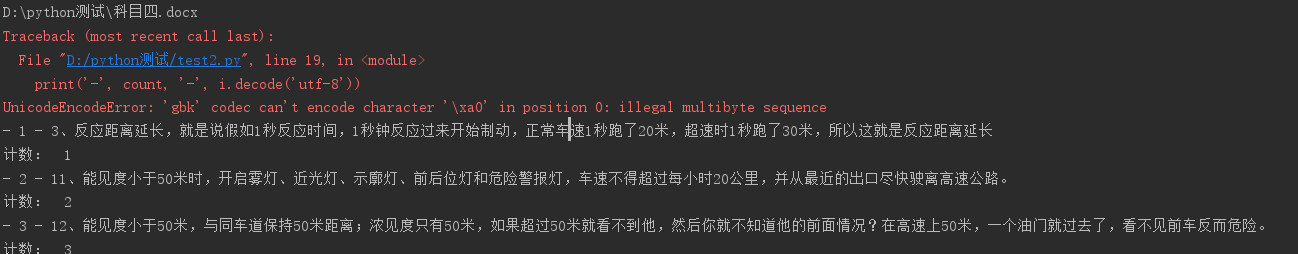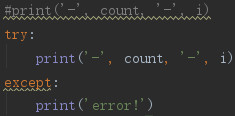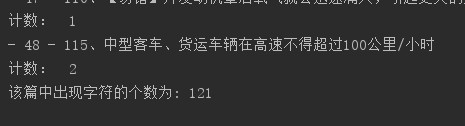python3 word="0"的编码如何变为“utf-8”

python3 最近要通过python实现搜索文件中的关键词出现次数的功能，定义输入的关键字字符串为word=""，代码从docx文件读取编码为"utf-8"，然后进行匹配搜索。目前输入word="1",word="0"都会报错，word="1234"就不会报错，分析大概是**编码问题**导致的。总的来说，我希望检索“0”这个字符串在某个word文档中出现的次数；是需要从word加载的内容全部转为unicode或者utf-8编码再匹配查找吗？目前“0”会在循环的某个判断停掉 ：if i.find(word) != -1:，关于在匹配关键词时用什么编码这块比较小白，希望大神可以帮忙看下：

``````# -*- coding: UTF-8 -*-
from docx import Document
import re, chardet
filename = "D:\python测试\科目四.docx"
word = "米".encode(encoding='utf-8')
#打开文档
document = Document(filename)
print (filename)
#读取每段资料
l = [paragraph.text.encode(encoding='utf-8', errors='ignore') for paragraph in document.paragraphs]
count = 0
count_2 = 0
j = 0
for i in l:
i = i.strip()
if i.find(word) != -1:
count = count + 1
j = j + 1
print('-', count, '-', i.decode('utf-8'))
print("计数： ", j)
count_2 = count_2 + j
print("该篇中出现字符的个数为:", count_2)
``````############################2个回答

# -*- coding: UTF-8 -*-

from docx import Document
import re, chardet
filename = "D:\python测试\科目四.docx"
word = "米"
#打开文档
document = Document(filename)
print (filename)
#读取每段资料
l = [paragraph.text for paragraph in document.paragraphs]
count = 0
count_2 = 0
j = 0
for i in l:
i = i.strip()
if i.find(word) != -1:
count = count + 1
j = j + 1
print('-', count, '-', i)
print("计数： ", j)
count_2 = count_2 + j
print("该篇中出现字符的个数为:", count_2)MatrixAaron 回复: 刚才又改了下：#print('-', count, '-', i) try: print('-', count, '-', i) except:
6 个月之前 回复MatrixAaron 刚才又改了下：
6 个月之前 回复MatrixAaron 没问题，但是当word="0"时最后一个print没输出，其他情况都是对的，这个如何解释
6 个月之前 回复
``````"0"的ascii是48，"\0"才是0，你到底是要什么编码。你可以用ord/chr在数字和对应的ascii之间转换。

``````MatrixAaron 总的来说，我希望检索“0”这个字符串在某个word文档中出现的次数；是需要全部转为unicode或者utf-8编码再匹配查找吗；目前“0”会在循环末尾在这个判断停掉 ：if i.find(word) != -1:，关于在匹配关键词时用什么编码这块比较小白，可以讲一下吗，谢谢
6 个月之前 回复